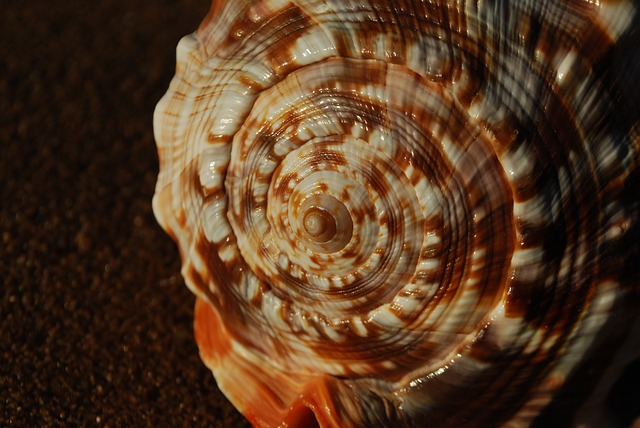# Fibonacci Sequence

The Fibonacci sequence is a very special mathematical sequence that begins with the numbers 0 and 1. Every subsequent number is found by adding the two numbers that are before it, and the sequence can be calculated indefinitely.

Adding 0 and 1 together make 1, so the third item in this sequence is just 1 again. 1 and 1 make 2, so that’s the fourth item in the sequence. The first 10 numbers are 0, 1, 1, 2, 3, 5, 8, 13, 21, and 34; check them to see that each number is the sum of the two preceding numbers!

## Summarizing the Fibonacci Sequence

To describe this sequence in math notation, all we have to do is write:
x0 = 0
x1 = 1
Xn = xn – 1 + xn – 2 for all integers n > 1.

## The Fibonacci Sequence in NatureDraw squares with the widths from the Fibonacci sequence (1, 1, 2, 3, 5, 8, 13, 21 to start with), and you can build a beautiful spiral. This is the spiral we see in the Milky Way and on shells such as the nautilus.But Fibonacci spirals aren’t the only place this special mathematical sequence shows its head. The branching of leaves in stems and branches from a central trunk often follow Fibonacci patterns. Flower pistils, like those in this chamomile, are also arranged in Fibonacci patterns as well.## The Fibonacci Sequence and the Golden Ratio

A n approaches infinity, the ratio of two successive Fibonacci numbers xn/xn – 1 approaches the golden ratio. This is a special ratio that you’ll often come across if you take ratios in simple geometric figures and turns out to be the ratio of width and height of the rectangle most pleasing to the human eye.

## References

McGraw, Victoria. Seven Beautiful Examples of the Fibonacci Seq. in Nature. Retrieved from https://www.theodysseyonline.com/7-beautiful-examples-fibonacci-sequence-nature on November 17, 2019

CITE THIS AS:
Stephanie Glen. "Fibonacci Sequence" From StatisticsHowTo.com: Elementary Statistics for the rest of us! https://www.statisticshowto.com/fibonacci-sequence/